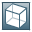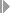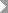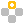Show TOC

###Tax Formula - Setup Window Operation Field: Brazil and IndiaUse this field in the Tax Formula - Setup window to select valid operations for the tax formula.

To open the window, chooseAdministrationSetupFinancialsTax Engine Configuration.

##### Arithmetic Operations

Operation

Description

Example

Result

+

X=2

X=X+1

3

Subtraction

X=2

X=5-X

3

*

Multiplication

X=4

X=X*5

20

/

Division

X=5

X=15/X;

X=5

X=X/2

3;

2.5

%

Modulus (division remainder)

5%2;

10%8;

10%2;

1;

2;

0

(,)

Priority

5*(2+3)

25

Round (Number, Decimals as Number)

A predefined function for rounding.

The rounding rules follow these settings:

• Rounding Method field in the Document Settings window

• Rounding field in the Define Currencies window

• Decimal Place field in the General Settings window, Display tab

Round (2.134,2);

Round (2.-1)

2.13;

0

Round (Number, Type)

A predefined function for rounding.

You can choose only one of the following types:

• Percents

• Prices

• Amounts

• Quantities

The rounding rules follow the settings of the Decimal Place field in the General Settings window, Display tab.

Round (2.134, Amounts)

If the setting of the Decimal Place Amounts field is 2.

2.13

##### Condition Operations

Operations

Description

Example

Result

<

Less than

5<8

True

<=

Less than or equal to

5<=8

True

==

Equal to

5==8

False

!=

Not equal

5!=8

True

>=

Greater than or equal to

5>=8

False

>

Greater than

5>8

False

If…else

Condition operate

if (X<6) {X=X+2} else

{X=X+1}

X=3

5Example

ICMS

Industrialization or Reselling or Consumption or Assets

ICMS Gross Amt = Item Net Value / (1-((ICMS rate/100)*(Base/100)))

ICMS Tax Amount = ICMS Gross Amount – Net Value

Base Amount = Item Net Value / (1-((ICMS rate/100)*(Base/100))*(1+(IPI rate/100)*(IPI Base/100)))

ICMS Amount = Base Amount * ((100-ICMS rate)/100)*(Base/100))

End of the example.
##### Grammar Rules

The language is case-sensitive.

1. Solo expressions are not supported. Only statements are valid.

Valid statements include:

• Assignment

• If

• While

• Compound

2. Rules for assignment statements:

1. The referred local variable must be initialized.

The following statements are invalid:

a = a + 1

/*the local variable 'a' is not assigned a value before */

b = a

/*the local variable 'a' is not assigned a value before */

2. The referred external local variable in the block cannot be assigned a value with a different type.

The following statements are incorrect:

a = "124"

/*it is not correct because the type of the local variable 'a' is string, which can be determined by the latest statement before the block*/

3. Rules for if / while statements:

1. The type of the expression enclosed within "(" and ")" must be of type boolean:

1. True

2. False

3. The result of the relationship expression.

2. The statements enclosed within "{" and "}" plus "{" and "}" consist of a block, so rule 2.b) is applicable.

4. Rule for functions:

1. This release supports only the "Round" function, which has two types of prototypes:

• Round (Number, Decimals as Number)

/* The first parameter "number" includes integer and real and represents the rounding number; the second parameter "integer" includes integer type and only represents the digitals after the decimal point */

• Round (Number, Type)

/* The first parameter is the same as the first prototype; the value of the second parameter "type" must be one of the following values: Percents, Prices, Amounts, or Quantities */

5. Rules for expressions:

1. Logic expression:

Operators: && > ||

Operands: value must be boolean

2. Relationship expression:

Operators: >, >=, ==, !=, <, <=

Operands:

• If there is one string value between two operands, the non-string value is changed to the corresponding string value before a comparison is made

• If there is no string value between two operands, the values of integer, real and boolean can be comparedExample

• The value of ("truf" > true) is true. Here the second operand is converted to "true".

• The value of (2 < "12") is false. Here the first operand is converted to "2".

• The value of (2< true) is false. Here the second operand is converted to 1.

• The value of (2>false) is true. Here the second operand is converted to 0.

End of the example.
3. Arithmetic expression:

Operators: (*, /, %) > (+, -)

Operands:

• The operands for "%" must be integer type\

• The operands for the operators, except "%" and "+" , must be non-string type, including integer, real and boolean

• The operands for "+" can be any type. If there is a string value of the operands, the result will be string; otherwise, the result will be numeric.

• If two operands are both integer, the result of "/" will also be integer. For example, 2 / 4 = 0 (divide exactly)

4. Unary expression:

Operators: !, -

Operands:

• For "!", it must be boolean value

• For "-", it must be non-string value

5. Bracket expression: the highest priority expression

Operators: (, )

Their priority is: 1) < 2) < 3) < 4) < 5)

6. Other rules:

1. Strings cannot be assigned to the non-string output parameter

2. You cannot assign a value to the input parameter

3. Number overflow - when you exceed the maximum value 9223372036854

4. All output variables must be assigned values

##### Reserved Words (case sensitive)
 if Percents ContinueNotice Round else Prices ContinueNextPageNotice while Amounts B1Notice True Quantities false
 COMPILER B1 CHARACTERS letter = "ABCDEFGHIJKLMNOPQRSTUVWXYZabcdefghijklmnopqrstuvwxyz" . digit = "0123456789" . cr = CHR(13). tab = CHR(9) . If = CHR(10) . anyButQuote = ANY – “ ' ” anyButApostrophe = ANY – “ ' ” COMMENTS FROM "/*" TO "*/" IGNORE cr + lf + tab TOKENS ident = letter {letter | digit | '_'} . number = digit {digit}. real = digit {digit} "." digit {digit}. string = '"' {anyButQuote} '"' | "'" {anyButApostrophe} "'". PRODUCTIONS B1 = Body. Body = StatSeq . StatSeq = Stat [";"] {Stat [";"]}. CompoundStat = "{" StatSeq "}". Stat = AssignStat | IfStat | WhileStat | CompoundStat. AssignStat = Ident "=" Exp. IfStat = "if" "(" Exp ")" CompoundStat [ "else" CompoundStat ]. WhileStat = "while" "(" Exp ")" CompoundStat. Exp = ConExp. ConExp = OrExp. OrExp = AndExp { "||" AndExp }. AndExp = RelExp { "&&" RelExp }. RelExp = AddExp [ RelOp AddExp]. RelOp = ( "<" | "<=" | ">" | ">=" | "==" | "!=" ). AddExp = MulExp { AddOp MulExp}. AddOp = ( "+" | "-" ). MulExp = Factor { MulOp Factor }. MulOp = ( "*" | "/" | "%" ). Factor = ("true" | "false" | integer | string | real |"(" Exp ")" | IdentOrFunction | Enum | UnaryOp Factor). IdentOrFunction = Ident (ParamList | ). Ident = ident. ParamList = "(" [Exp { "," Exp}] ")". Enum = RoundTypeEnum | SystemStringEnum. RoundTypeEnum = ("Percents" | "Prices" | "Amounts" | "Quantities"). SystemStringEnum = ("ContinueNotice" | "ContinueNextPageNotice" | "B1Notice"). UnaryOp = ("-" | "!"). END B1.